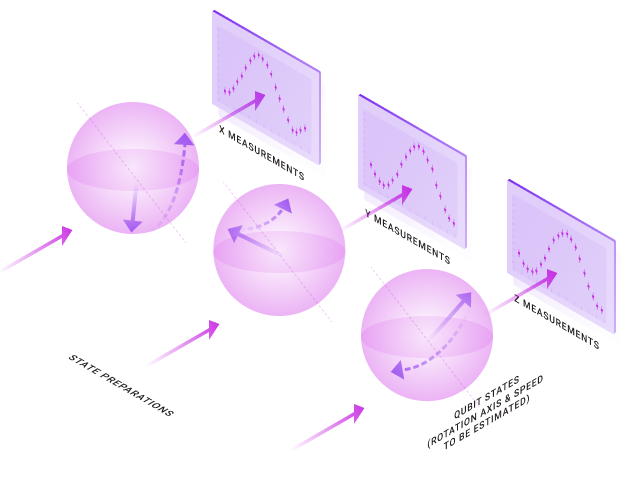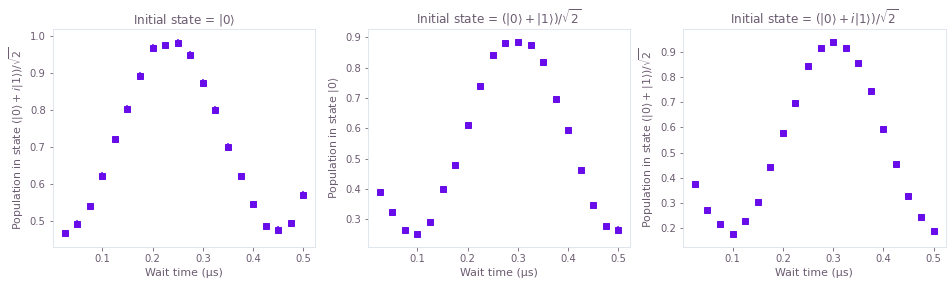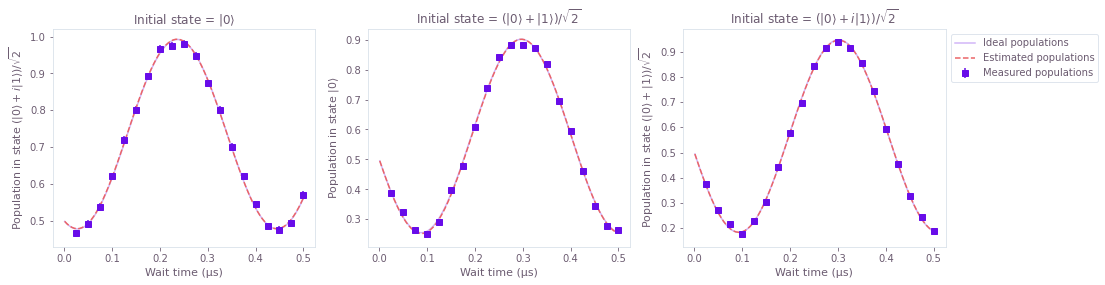# How to perform Hamiltonian parameter estimation using a small amount of measured data

Estimate Hamiltonian model parameters using measured data and the graph-based optimization engine

The Q-CTRL Python package optimization engine provides a large, modular collection of configuration options that allows it to be used for a range of tasks in quantum control, including estimating Hamiltonian parameters in a model using measured data.

In this notebook, we show how this engine can be used to determine the parameters that characterize aspects of a hardware system using a small amount of measured data. You can read our Characterizing your hardware using system identification in Boulder Opal topic to learn more about parameter estimation, as well as which optimization engine to use depending on your system size or the amount of measured data you have.

## Summary workflow for Hamiltonian parameter estimation

### 1. Perform measurements to probe the system

In general the output of probe measurements will be estimates of various parameters, such as entries in a tomographic reconstruction of a state. Suitable probe pulses must be crafted which give access to terms relevant to a model being captured in order to constrain the optimization search space.

### 2. Build a graph-based optimization encoding the problem

Represent the cost function to be minimized using a graph. Define optimization parameters in the graph using qctrl.operations.optimization_variable. Execute the optimization using qctrl.functions.calculate_optimization by assigning the optimization variables to output nodes of the graph.

## Worked example: Identifying constant terms of a single-qubit Hamiltonian

In this example, we will consider how to estimate a constant Hamiltonian that rotates a single qubit. In this case, preparing different initial states and letting the qubit evolve for different times before measuring it will reveal information about the parameters of the qubit rotation. This setup is illustrated below.In more detail, if we exclude terms proportional to the identity (which affect all states in the same way and therefore are irrelevant to the qubit's evolution), a constant single-qubit Hamiltonian can be written in terms of the Pauli matrices:

$$H = \frac{1}{2} \left( \Omega_x \sigma_x + \Omega_y \sigma_y + \Omega_z \sigma_z \right).$$

The task of the optimizer in this case will be to determine these three parameters $\Omega_x$, $\Omega_y$, and $\Omega_z$. This can be done by preparing the system in different initial states, and then letting it evolve for different wait times, before measuring it. The sets of measured points are then provided to the optimization engine, which finds the parameters most likely to have generated that series of points.

In this example we will rely on simulated measurement data which we generate first. The experiments used here to determine a constant term in the Hamiltonian consist of simply letting a qubit evolve for different initial states, during different wait periods. We prepare these qubits in the eigenstates of $\sigma_z$, $\sigma_x$, and $\sigma_y$, and then measure the populations in eigenstates of $\sigma_y$, $\sigma_z$, and $\sigma_x$, respectively. Information about the evolution of these three initial states is sufficient to characterize the three parameters that form the Hamiltonian. Moreover, measuring populations that are not in the same eigenbasis as the initial states allows us to get information about the direction of the rotation of the qubit, depending on whether the population initially increases or decreases.

import matplotlib.pyplot as plt
import numpy as np
from qctrlvisualizer import get_qctrl_style
from scipy.linalg import expm

from qctrl import Qctrl

plt.style.use(get_qctrl_style())

# Define standard matrices
sigma_x = np.array([[0, 1], [1, 0]], dtype=complex)
sigma_y = np.array([[0, -1j], [1j, 0]], dtype=complex)
sigma_z = np.array([[1, 0], [0, -1]], dtype=complex)

# Start a session with the API
qctrl = Qctrl()

# Define parameters to be estimated
actual_Omega_x = (2.0 * np.pi) * 0.5e6  # Hz
actual_Omega_y = (2.0 * np.pi) * 1.5e6  # Hz
actual_Omega_z = (2.0 * np.pi) * 1.8e6  # Hz

actual_Omegas = [actual_Omega_x, actual_Omega_y, actual_Omega_z]

# range of wait times in the different experiments
wait_times = np.linspace(25e-9, 500e-9, 20)

# list of initial states
initial_states = np.array(
[
[[1.0 + 0.0j], [0.0 + 0.0j]],
[[1.0 + 0.0j], [1.0 + 0.0j]] / np.sqrt(2.0),
[[1.0 + 0.0j], [0.0 + 1.0j]] / np.sqrt(2.0),
]
)
initial_state_names = [
r"$\vert 0 \rangle$",
r"$(\vert 0 \rangle + \vert 1 \rangle)/\sqrt{2}$",
r"$(\vert 0 \rangle +i \vert 1 \rangle)/\sqrt{2}$",
]

# list of states whose population will be measured at the end
projector_states = np.array(
[
[[1.0 + 0.0j], [0.0 + 1.0j]] / np.sqrt(2.0),
[[1.0 + 0.0j], [0.0 + 0.0j]],
[[1.0 + 0.0j], [1.0 + 0.0j]] / np.sqrt(2.0),
]
)
projector_state_names = [
r"$(\vert 0 \rangle +i \vert 1 \rangle)/\sqrt{2}$",
r"$\vert 0 \rangle$",
r"$(\vert 0 \rangle + \vert 1 \rangle)/\sqrt{2}$",
]

# define Hamiltonian
Hamiltonian = lambda Omegas: 0.5 * (
Omegas * sigma_x + Omegas * sigma_y + Omegas * sigma_z
)

# define unitaries for Hamiltonian
Ut = lambda t, Omegas: expm(-1.0j * t * Hamiltonian(Omegas))

# define function to calculate the populations given Omegas and wait times
# this function first calculates the unitaries for all wait times and then
# calculates the projected populations for different initial states and projectors.
# the return value is thefore in the shape of [len(projector_states), len(wait_times)]
def get_populations(Omegas, wait_times):
unitaries = np.array([Ut(t, Omegas) for t in wait_times])
return np.array(
[
np.abs(
np.matmul(
projector_state.conj().T, np.matmul(unitaries, initial_state)
).flatten()
)
** 2
for projector_state, initial_state in zip(projector_states, initial_states)
]
)

# calculate expected values
expectation_values = get_populations(actual_Omegas, wait_times)

# actual measurement results will have a certain uncertainty to them
# we will estimate the standard deviation of this error at 0.01
measurement_errors = 0.01 * np.ones_like(expectation_values)
measurement_results = np.random.normal(loc=expectation_values, scale=measurement_errors)

# plot inputs
measurement_times = wait_times * 1e6

fig, axs = plt.subplots(1, len(projector_states), figsize=(16, 4))
for n in range(len(projector_states)):
axs[n].set_title("Initial state = " + initial_state_names[n])
axs[n].set_ylabel("Population in state " + projector_state_names[n])
axs[n].set_xlabel("Wait time (µs)")
axs[n].errorbar(
measurement_times,
measurement_results[n],
yerr=measurement_errors[n],
fmt="s",
color="C0",
)The graphs above show the values of the measurement results, considering an error whose standard deviation is 1% of the population. Each graph represent a set of experiments with the same initial state (given in the title) and same measured population (given in the $y$ axis). The error bars in the graphs have a length of $2\sigma$, or 95% reliability.

### Estimating the parameters of the Hamiltonian

The parameter estimation for the Hamiltonian terms is performed by finding the form of the evolution that most closely matches the measured data points. The optimization will seek to minimize a cost function parameterized by $\Omega_x$, $\Omega_y$, and $\Omega_z$:

$$C = \sum_i \frac{[P_i-p_i(\Omega_x, \Omega_y, \Omega_z)]^2}{2(\Delta P_i)^2}\,.$$

Doing so returns the best choice of parameters that generates the original dynamics of the system, and also allows us to calculate the precision of the estimated parameters. This is done by using the Cramér–Rao bound to identify the Hessian of the cost function with the inverse of the covariance matrix for the variables estimated.

with qctrl.create_graph() as graph:
# forbids frequencies whose half-periods are shorter than the smaller spacing between points

# Parameters to be estimated
Omegas = [
qctrl.operations.optimization_variable(
1,
lower_bound=-1.0 / min_time_resolution,
upper_bound=1.0 / min_time_resolution,
name=name,
)
for name in ["omega_x", "omega_y", "omega_z"]
]

# Create coefficients of the Hamiltonian
# to calculate the infidelities at the intermediate times, you can create a batch of Hamiltonian
# coefficients with the duration set to 1 and signal value set to be scaled by the intermediate times
signals = [
qctrl.operations.pwc_signal(duration=1, values=omega * wait_times[:, None])
for omega in Omegas
]

hamiltonian = qctrl.operations.pwc_sum(
[
signal * operator
for signal, operator in zip(
signals, [sigma_x / 2, sigma_y / 2, sigma_z / 2]
)
]
)

# Calculate the projected populations
populations = [
1
- qctrl.operations.infidelity_pwc(
hamiltonian=hamiltonian,
target=qctrl.operations.target(
operator=projector_state @ initial_state.T.conj()
),
)
for projector_state, initial_state in zip(projector_states, initial_states)
]

# Calculate cost
cost = qctrl.operations.sum(
[
qctrl.operations.sum(
(population - measurement_result) ** 2.0
/ (2.0 * measurement_error ** 2.0)
)
for measurement_result, measurement_error, population in zip(
measurement_results, measurement_errors, populations
)
],
name="cost",
)

# Calculate Hessian
hessian = qctrl.operations.hessian_matrix(cost, Omegas, name="hessian")

# Estimate the parameters
result = qctrl.functions.calculate_optimization(
cost_node_name="cost",
output_node_names=["omega_x", "omega_y", "omega_z", "hessian"],
graph=graph,
)

estimated_Omegas = [
result.output["omega_x"]["value"],
result.output["omega_y"]["value"],
result.output["omega_z"]["value"],
]

# Calculate 2-sigma uncertainties (error bars give 95% precision)
hessian = result.output["hessian"]["value"]
uncertainties = 2.0 * np.sqrt(np.diag(np.linalg.inv(hessian)))
Omega_x_uncertainty, Omega_y_uncertainty, Omega_z_uncertainty = uncertainties

Your task calculate_optimization has started.


# Now we verify the estimation results using a different set of wait times
wait_times_verification = np.linspace(1e-9, 500e-9, 100)

# calculate expected values for verification
expectation_values_verification = get_populations(
actual_Omegas, wait_times_verification
)

# calculate estimated values for verification
estimated_values_verification = get_populations(
estimated_Omegas, wait_times_verification
)

# Plot results
measurement_times_verification = wait_times_verification / 1e-6

fig, axs = plt.subplots(1, len(projector_states), figsize=(16, 4))
for n in range(len(projector_states)):
axs[n].set_title("Initial state = " + initial_state_names[n])
axs[n].set_ylabel("Population in state " + projector_state_names[n])
axs[n].set_xlabel("Wait time (µs)")
axs[n].errorbar(
measurement_times,
measurement_results[n],
yerr=measurement_errors[n],
fmt="s",
label="Measured populations",
color="C0",
)
axs[n].plot(
measurement_times_verification,
expectation_values_verification[n],
color="C0",
label="Ideal populations",
alpha=0.3,
)
axs[n].plot(
measurement_times_verification,
estimated_values_verification[n],
"--",
label="Estimated populations",
color="C1",
)
axs[-1].legend(loc=2, bbox_to_anchor=(1, 1))

# print parameter estimates
print("real Omega_x =", actual_Omegas * 1e-6, "MHz")
print(
"estimated Omega_x = (",
np.around(estimated_Omegas * 1e-6, 3),
"±",
np.around(Omega_x_uncertainty * 1e-6, 3),
") MHz",
)

print("real Omega_y =", actual_Omegas * 1e-6, "MHz")
print(
"estimated Omega_y = (",
np.around(estimated_Omegas * 1e-6, 3),
"±",
np.around(Omega_y_uncertainty * 1e-6, 3),
") MHz",
)

print("real Omega_z =", actual_Omegas * 1e-6, "MHz")
print(
"estimated Omega_z = (",
np.around(estimated_Omegas * 1e-6, 3),
"±",
np.around(Omega_z_uncertainty * 1e-6, 3),
") MHz",
)

real Omega_x = 3.1415926535897927 MHz
estimated Omega_x = ( 3.093 ± 0.102 ) MHz
real Omega_y = 9.42477796076938 MHz
estimated Omega_y = ( 9.47 ± 0.135 ) MHz
real Omega_z = 11.309733552923253 MHz
estimated Omega_z = ( 11.279 ± 0.117 ) MHzThe three parameters estimated above, $\Omega_x$ (Omega_x), $\Omega_y$ (Omega_y), and $\Omega_z$ (Omega_z) correspond to the three coefficients present in the single-qubit Hamiltonian given above. The errors in the parameter estimates correspond to 2 times the standard deviation and thus have 95% reliability.

In the graphs above, we can compare the curves that represent the populations that would be measured if the system followed the estimated Hamiltonian (dashed), and the populations that would be measured if perfect measurements were performed on the real Hamiltonian (solid). Each graph corresponds to sets of experiments with a given initial state and final measurement, as indicated in the title and $y$ label of each of them. The close match between the two curves highlights the fact that the estimated parameters are close to the real values.